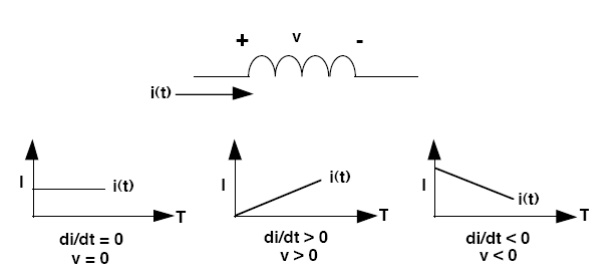Home | | Linear Integrated Circuits | Switching Fundamentals

# Switching Fundamentals

The law of inductance: If a voltage is forced across an inductor, a current will flow through that inductor (and this current will vary with time).

Switching Fundamentals

The law of inductance

If a voltage is forced across an inductor, a current will flow through that inductor (and this current will vary with time). The current flowing in an inductor will be time-varying even if the forcing voltage is constant. It is equally correct to say that if a time-varying current is forced to flow in an inductor, a voltage across the inductor will result. The fundamental law that defines the relationship between the voltage and current in an inductor is given by the equation:

v = L (di/dt)

Two important characteristics of an inductor that follow directly from the law of inductance are:

1)       A voltage across an inductor results only from a current that changes with time. A steady (DC) current flowing in an inductor causes no voltage across it (except for the tiny voltage drop across the copper used in the windings).

2)       A  current flowing in an inductor can not change value instantly (in zero time), as this would require infinite voltage to force it to happen. However, the faster the current is changed in an inductor, the larger the resulting voltage will be. Note: Unlike the current flowing in the inductor, the voltage across it can change instantly (in zero time). The principles of inductance are illustrated by the information contained in Figure.The important parameter is the di/dt term, which is simply a measure of how the current changes with time. When the current is plotted versus time, the value of di/dt is defined as the slope of the current plot at any given point. The graph on the left shows that current which is constant with time has a di/dt value of zero, and results in no voltage across the inductor.

The center graph shows that a current which is increasing with time has a positive di/dt value, resulting in a positive inductor voltage. Current that decreases with time (shown in the right-hand graph) gives a negative value for di/dt and inductor voltage. It is important to note that a linear current ramp in an inductor (either up or down) occurs only when it has a constant voltage across it.

Study Material, Lecturing Notes, Assignment, Reference, Wiki description explanation, brief detail
Linear Integrated Ciruits : Application of ICs : Switching Fundamentals |